Your browser does not support the HTML5 canvas tag.
Domain
Range

# JamworksPro Takes Online Graphing Seriously

Jaye at JamworksPro has been busy over the past few months polishing his Flagship Multivariate Algebraic Expression Based Graphing Calculator, Math Dynamics. It’s more than just a graphing calculator. It’s an entire Mathematics Management System.

Warrenton Oregon, Oct 28, 2021— From the JamworksPro R&D Department Comes Math Dynamics, the Online Arithmetic Machine (https://mathdynamics.net). Math Dynamics includes both an Android App as well as it’s Complementary Web App. At the core of Math Dynamics is the ability to write mathematical expressions using most nearly any string of alphanumeric characters as variables.

“Math doesnt have to be cryptic”

a*x^2 + b*x + c
can also be written as
ace*xray^2 + baker*xray + charlie

Math Dynamics keeps it simple with a straight forward approach to variables, constants and the operators used to form mathematical expressions.

If  h is the height measured in feet,  t  is the number of seconds the object has fallen from an initial height h0 with an initial velocity or speed v0 (ft/sec), then the model for height of a falling object is:

h(t)=−16 * t^2 + v0 * t + h0

“Free Form Variable Names make more sense in the physical world”

height(elapsedTime) = -16 * elapsedTime^2 + initialVelocity * elapsedTime + initialHeight

Mathematical Expressions are packaged in Math Dynamics as Function Definition Cards. The Variables are listed in a table that allows their values to be edited. Any one of the variables can be used as an independent variable to plot the graph of the function. By entering start, end and increment values in that order will define that variable as independent and will plot a graph accordingly. Function Definition Cards can be named and saved as .xg2 files for portablility. These .xg2 files can be opened by both the Web App as well as the Android App.

Collections of Function Cards are packaged in the Math Dynamics Pallet (https://mathdynamics.net/pallet.html). Both the Android App and the Web App have a Pallet. Function Definition Cards are easily added to or removed from the Pallet. The Pallet in the Android App supports Function Definition Cards that each have a self contained graph while the Pallet in the Web App supports a single graph on which any of the Function Definition Cards may use to plot its graph.

The Pallet in the Web App is very powerful. It supports plotting multiple functions on the same graph. Each plot can have a different color and each plot can also be hidden. Like Function Definition Cards, Pallets can be named and can be downloaded and saved for portability.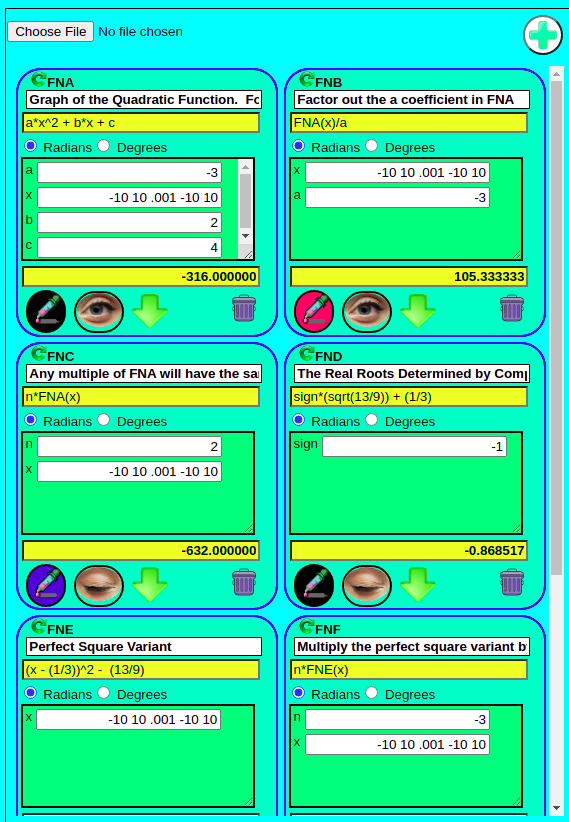The Web App Pallet supports up to 26 custom functions. Each function can be plotted on the common graph. The visibility of the graph can be turned on or off. Each graph can have a custom color assigned to it.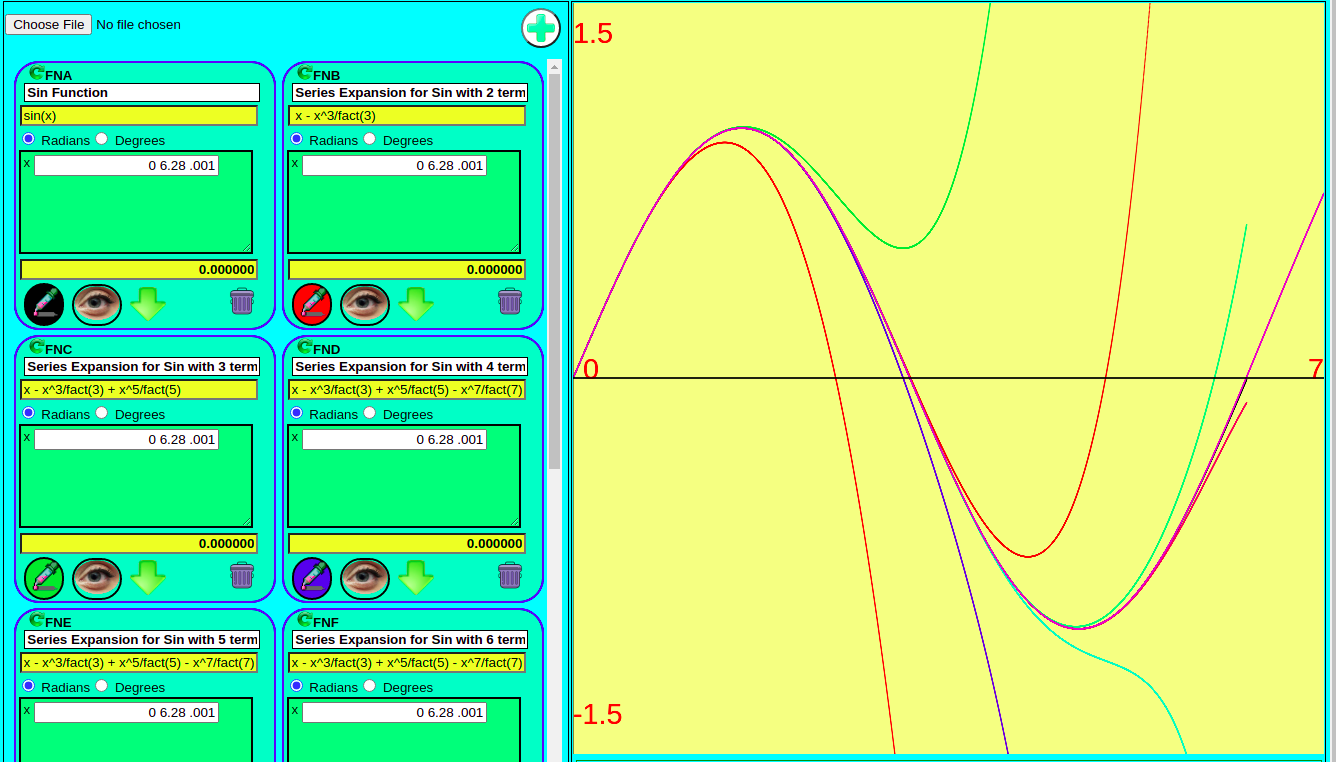This Graph of the Maclaurin Series to calculate the sin of an angle demonstrates the ability to plot multiple functions on the same graph.

# Exploring the Maclaurin Series Expansion sin(x)

sin(x) = $$x – x^3/3! + x^5/5! – x^7/7! + x^9/9! –$$…

The sin(x) is plotted in black.

Lets break down the amount of work we need to do depending on size of the angle.

2 Terms are needed for angles 0 through .9 radians

3 Terms are needed for angles >.9 and <1.57

4 Terms are needed for angles >1.57 and <2.5 radians

5 Terms are needed for angles >2.5 and <3.3 radians

6 Terms are needed for angles >3.3 and <4 radians

7 Terms are needed for angles >4 and <4.5 radians

8 Terms are needed for angles >4.5 and <5.5 radians

9 Terms are needed for angles >5.5 and <=2*pi radians

This can be A LOT of WORK with pencil and paper.

Fortunately, the sin of any (A)NGLE > 2*pi will be identical to the sin of an (a)ngle less than or equal to 2*pi.

So for example, given an (A)ngle of 31.72 radians its corresponding (a)ngle will be .304

n = int(A/(2*pi))
a = A-2*n*pi

Given A = 31.72 then n = 5
hence a = 31.72-(2*5*pi)= .304

GREAT!! we only need 2 Terms x – x^3/fact(3)

This interactive Math Dynamics Pallet demonstrates how the Maclaurin Expansion Series can be used to calculate the sin of any angle

# A Quadratic Deep Dive into Real Roots

The Math Dynamics Multivariable Algebra Expression Based Graphing Calculator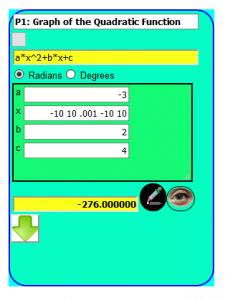This Math Dynamics Function Card will generate a plot from -10 to 10 in increments of .001 and limit the Y axis from 10 to -10

Complete the Square method is an algorithm to find the Roots of a Quadratic Equation both Real and Complex.  This exercise focuses on using the “Complete the Square” method to calculate the Real Roots so it only makes sense to use a Quadratic Function that has two real roots.

$$-3*x^2 + 2*x + 4$$

The plot of this function visually demonstrates that this Quadratic Function has 2 real roots.

The exact values of these two real roots can be calculated using the “Complete the Square” method.  This requires two things:

1. $$-3*x^2 + 2*x + 4 = 0$$
2. The $$a*x^2$$ term requires that a = 1, not -3

To resolve the second requirement requires that both sides of the quadratic equation be multiplied by -(1/3) such that the new Quadratic Function is:

$$x^2 -(2/3)*x -(4/3) = 0$$

Which produces a different graph.  This new graph shares the same roots as the original graph.

I found this to be a quite interesting property.  It appears though that all multiples of a Quadratic Function have equal roots

# JamworksPro Introduces Math Dynamics™ for Android

##Math Dynamics is more than a calculator. It’s a platform for managing math intensive information. From simple unit conversions to advanced algebraic and trigonometric analysis. Math Dynamics allows the User to craft custom Function Definitions from many discipline’s such as Statistics, Marketing, Engineering, etc.

Its a tool for Math. Another instrument that you can add to your tool chest that gives you an edge when it comes to everyday activities that demand a quick calculation.

Use Calculator mode for a quick calculation. Calculations can range from simple arithmetic to multivariate formulas like Cost per Unit, Miles per Gallon, etc..Any formula created in the Calculator can be saved to the Pallet.

The Pallet is a collection of Function Definition Cards. Each card contains the function definition and a list of all of the variables defined and it’s evaluated result. Users can quickly evaluate any Function Definition simply by editing the values of the variables in the table. The result is evaluated in real time as you change the values of the variables.

With Math Dynamics you can share your function definitions with other users. Export and Import functions allow the Users to save and share any of the Function Definition’s in the Pallet as a Math Dynamics XML file. These files also include the values of the variables, the result and the Trig Mode at the time of Export.

# A Treatise on Trigonometry

Trigonometry is based on the fundamental principle of ONE length.  The length in this context is immaterial because with Trigonometry we only need be concerned about ONE.  For example if you draw a circle it is ONE circle no matter how big or small.  Because it is a circle it has only ONE center with a constant distance to the edge of the circle.  This constant distance is ONE and is called the Radius.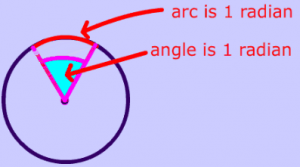The Unit Circle is fundamental to Trigonometry. Any circle has a constant Circumference that is exactly 3.14159… times its Diameter. The “units”, i.e. inches, centimeters, … are immaterial. Its radius is simply ONE unit.

Simple so far, just a circle with a center and a radius of ONE.  Now consider the radius as it sweeps through a small region of the circle forming a triangle with two sides of an equal length ONE  and forming an angle at the apex of the triangle.  An Angle is a unit that specifies the amount of the sweep of the radius that formed the triangle.

At this point we are finished with the circle.  It is no longer needed.  Also, our Triangle can now be moved and reoriented any way we wish.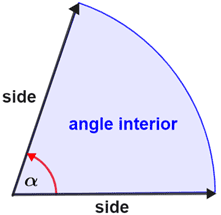It is imperative though that the lengths of the two radii forming the triangle remain equal and ONE.  The angle is most visually apparent when one of the sides of the Triangle lies along a horizontal axis.  At this point a perpendicular line can be drawn between the tip of one of radii and intersecting the other radii.  The perpendicular line intersects the radii  such that the length between the apex (angle)  and the intersection is a ratio of ONE.

Now, this is where it gets complicated.  Since the triangle has been conveniently aligned with one of the radii along the horizontal axis it can be said that the SIN is the distance (as a percentage of ONE) along a corresponding VERTICAL AXIS between the TIP of one radii and a HORIZONTAL AXIS.  Likewise the COS is the distance along a HORIZONTAL AXIS  between the TIP of one radii and a VERTICAL AXIS.